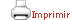Teaching plan for the course unit

 CloseGeneral information

Course unit name: Bayesian Statistics and Probabilistic Programming

Course unit code: 574184

Coordinator: Jose Fortiana Gregori

Department: Department of Mathematics and Computer Science

Credits: 3

Single program: S

 Estimated learning time Total number of hours 75

 Face-to-face and/or online activities 30
 -  Lecture with practical component Face-to-face 30 (Depending on sanitary circumstances, these sessions can be by distance video-meeting.)
 Supervised project 15
 Independent learning 30

 Competences to be gained during study

 CE5 - To know how to hypothesize and develop the intuition about a data set using exploratory analysis techniques. CE7 - To understand, develop and modify analytic and exploratory algorithms operating on data, being these tasks guided by a critical thought. CE8 - To be able to assess the validity of hypotheses by means of data analytics.

 Learning objectives
 Referring to knowledge Referring to knowledge: To master the Bayesian paradigm as the framework where assumptions and experimental evidences are quantitatively merged into a new outlook. To apply Bayesian thought to data modeling and prediction. To understand the rationale of and to know how to bring into practice Bayesian computations, mainly based on simulation and approximation. To be informed about and to be able to use ad hoc computer languages, specifically designed to handle random variables and their probability distributions.

 Teaching blocks

1. Probability, frequentist and subjective. Conditional probability. Bayes’s formula.

2. Random variables (r.v’s). Discrete and continuous r.v.’s. Pmf, pdf and cdf. Bivariate and multivariate distributions. Marginal distributions.

3. Bayes formula for r.v.’s. The Bayesian paradigm: likelihood, prior and posterior distributions.

4. Simulation. Random sequences (RS), uniform and non-uniform. Inverse cdf transformation. Acceptance-rejection method.

5. Probabilistic programming languages. BUGS/JAGS, Stan, Greta, Edward, PyMC.

6. Bayesian binomial model. Prior and posterior predictive distributions

7. Conjugate models. Normal model. Gamma-Poisson model. Dirichlet-Multinomial model. Prior choice. Maximum entropy priors.

8. Markov chains. Simulation of trajectories. Markov Chain Monte-Carlo (MCMC) methods. Metropolis-Hastings algorithm. Gibbs sampling. Slice sampling. Hamiltonian Monte Carlo (HMC)..

9. Linear regression. Logistic and Poisson regression. Other GLM’s.

10. Approximation methods: Laplace approximation, Variational inference.

11. Hierarchical Bayesian models.

 Teaching methods and general organization

 Sessions will combine short pieces of exposition by the instructor, mainly through illustrative examples, with application problems and hands-on treatment of code and data. It is not foreseen to have formal theoretical lectures. These sessions, depending on orders from the local health authority, can be either face-to-face or online at a distance. In the second case, appropriate materials will be published in advance, to facilitate a flipped classroom-style knowledge transmission. The division of students into groups will be adapted to this situation. Teaching will be structured as follows: Weekly, there will be an asynchronous one-hour session where the students autonomously work in the subject. Weekly, there will be a face-to-face one-hour session corresponding to theoretical-practical activities. If the health situation allows it, we will move to a face-to-face teaching style, keeping the hourly load remains at the same values, but classes will be held in face-to-face mode, and the distribution of students in groups can be varied to suit the face-to-face mode. When, and if, possible, students will be encouraged to organize themselves in small teams to deal with problem solving, including submission of home assignments or course project.

 Official assessment of learning outcomes

 Two homework assignments will be worth 60% of the final grade (30% each). If face to face sessions are possible, this component will be apportioned into class attendance and participation (15%) and homework submission (45%). A course project will be worth 40% of the final grade.   Examination-based assessment Two homework assignments will be worth 60% of the final grade (30% each).  A course project will be worth 40% of the final grade.

Book

Albert, J. (2009), Bayesian computation with R (Corr. 2nd printing edition). Springer.

Congdon, Peter D. (2019), Bayesian Hierarchical models with applications using R. CRC Press.

Davidson-Pilon, C. (2015), Bayesian Methods for Hackers: Probabilistic Programming and Bayesian Inference, Addison-Wesley.

Downey, A. (2014),  Think Stats. Probability and Statistics for Programmers. Second edition. Green Tea Press – O’Reilly.

Downey, A. (2013),  Think Bayes. Bayesian Statistics Made Simple.  Green Tea Press – O’Reilly.

Gelman, A., Carlin, J., Stern, H., Dunson, D., Vehtari, A. Rubin, D. (2014), Bayesian Data Analysis, Third edition. CRC Press.

Koduvely, H. (2015), Learning Bayesian Models with R. Packt Publishing.

Kruschke, J. (2015), Doing Bayesian Data Analysis: A Tutorial with R, JAGS, and Stan, 2nd Edition. Academic Press.

Martin, O. (2016), Bayesian Analysis with Python. Packt Publishing.

McElreath, R. (2020), Statistical rethinking - A Bayesian course with examples in R and Stan. Second edition. CRC Press.

Robert, C., Casella, G. (2010), Introducing Monte Carlo methods with R. Springer.